Article Index
Projectile Motion
Projectile Motion Continue
All Pages

PROJECTILE MOTION

We see one dimensional motion in previous topics. Now, we will try to explain motion in two dimensions that is exactly called “projectile motion”. In this type of motion gravity is the only factor acting on our objects. We can have different types of projectile type. For example, you throw the ball straight upward, or you kick a ball and give it a speed at an angle to the horizontal or you just drop things and make them free fall; all these are examples of projectile motion.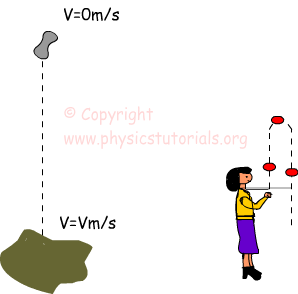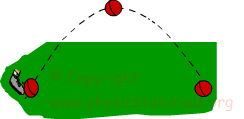In projectile motion, gravity is the only force acting on the object. I will explain this sentence with a picture and examples. First, look at the given picture which shows the motion path, velocities in different points and forces acting upon the object doing projectile motion.As you see in the picture given above, we have a projectile motion and velocity components at different positions. At the beginning the ball is thrown with an angle to the horizontal. V is its velocity and its direction is northeast. Vx and Vy are the X and Y components of our velocity. If we look at the forces acting on our ball we see only the gravity as a force. We examine our motion in two parts, first one is horizontal motion and second one is vertical motion. When we look at the horizontal motion of the object we see that it looks like example solved in free fall motion section. In vertical as you can see in the picture, our velocity is decreasing in the amount of gravitational acceleration. At the top where it reaches its maximum height vertical component of our velocity becomes zero as in the case of free fall examples. After Vy becomes zero our ball changes its direction and make free fall now. At the same levels magnitudes of Vy are the same however, their signs are opposite. Right side of our picture has “-“sign in front of the Vy because its direction is downward. Finally, when the ball hits the ground Vy reaches its beginning magnitude but opposite in direction. We see the effect of gravity on vertical motion. Now, let’s look at the horizontal part of our projectile motion. This part is so easy that you can understand from the picture, our horizontal component of velocity is constant during the motion. Why it is constant? What changes the velocity? In previous section we learned force concept that causes change in the state of motion. Look at our horizontal motion carefully. Is there any force acting on our object in horizontal direction +X or –X? The answer is actually no. However, in –Y direction gravity is acting on our object which makes Vy decrease and becomes zero at the top. All these explanations say that, we have two motions in projectile motion. One of them is constant motion in horizontal and other one is free fall under the effect of gravity in vertical. We tried to explain projectile motion with words. Now it is time to give equations of motion under two titles.

1. Vertical motion:

In vertical we said that gravity acts on our objects and give it negative acceleration “-9,8m/s²”. This means that, our velocity decreases -9,8m/s² in each second. We find the velocity of the free falling object by the equation V=g.t. If we have initial velocity then, our equation becomes;

V=Vit+gt where acceleration is -9,8m/s²

The distance in free fall is calculated by the equation;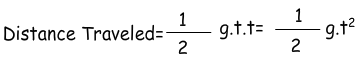As in the velocity case our distance is calculated considering the initial velocity of the object by the formula;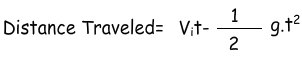We put “-“sign because direction of g is downward.

2. Horizontal motion:

We have constant motion in horizontal because there is no force acting on our object in horizontal direction. Thus, the X component of velocity is constant and acceleration in X direction is zero. The equation that is used to calculate distance and velocity is given below.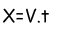You can find distance traveled, time elapsed from this equation.

Now I will solve some examples related to the each type of projectile motion.

Example In the given picture below, Alice throws the ball to the +X direction with an initial velocity 10m/s. Time elapsed during the motion is 5s, calculate the height that object is thrown and Vy component of the velocity after it hits the ground.Example John kicks the ball and ball does projectile motion with an angle of 53º to horizontal. Its initial velocity is 10 m/s, find the maximum height it can reach, horizontal displacement and total time required for this motion. (sin53º=0, 8 and cos53º=0, 6)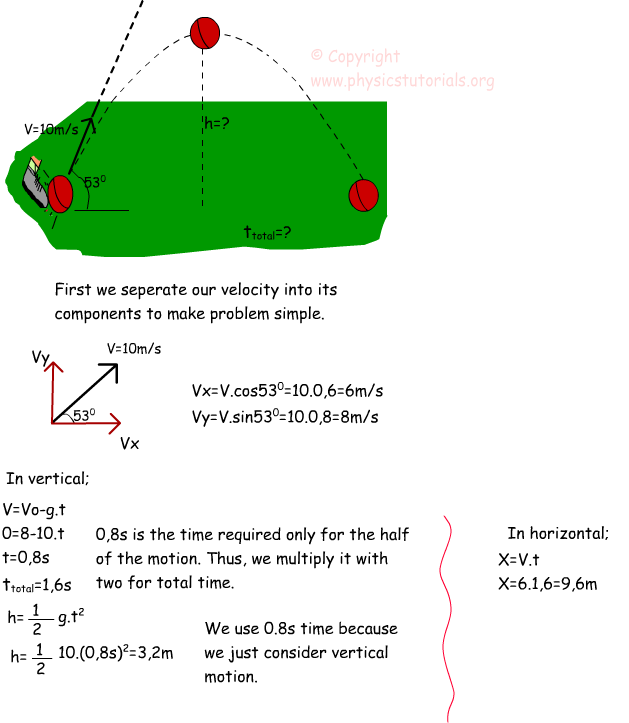Example In the given picture you see the motion path of cannonball. Find the maximum height it can reach, horizontal distance it covers and total time from the given information. (The angle between cannonball and horizontal is 53º and sin53º=0, 8 and cos53º=0, 6)Kinematics Exams

Related